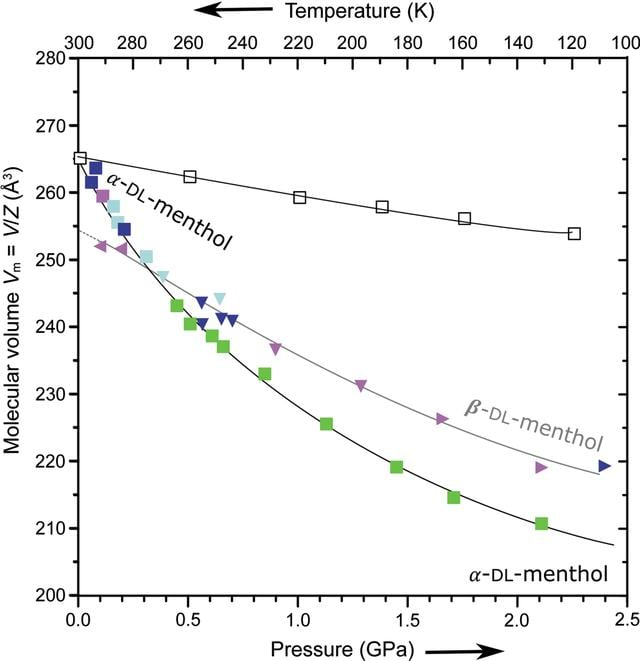disable zoom     view article Figure 5 Pressure (full symbols) and temperature (open squares) dependence of molecular volume (Vm = V/Z) for α-DL-menthol (squares) obtained at atmospheric pressure (green squares); and recrystallized up to 0.40 GPa from methanol:water solution (blue), ethanol:water solution (cyan) as well as from the pure melt (violet); all recrystallizations above 0.40 GPa yielded β-DL-menthol (triangles down), which also persisted after further compression (triangles right) and decompression below 0.40 GPa (triangles left). All estimated standard deviations are smaller than the plotted symbols. The least-squares functions (plotted lines) fitted to the experimental points are Vα(p) = 265.0 (4) − 52 (1)p + 18 (2)p2 − 2.5 (6)p3 and Vβ(p) = 255 (2) − 21 (6)p − 1(7)p2 + 1(2)p3 (Å3).

IUCrJ
Volume 10| Part 3| May 2023| Pages 341-351
ISSN: 2052-2525